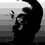# WooHooCreate a math problem based on this gif.Note by Lew Sterling Jr
5 years, 8 months ago

This discussion board is a place to discuss our Daily Challenges and the math and science related to those challenges. Explanations are more than just a solution — they should explain the steps and thinking strategies that you used to obtain the solution. Comments should further the discussion of math and science.

When posting on Brilliant:

• Use the emojis to react to an explanation, whether you're congratulating a job well done , or just really confused .
• Ask specific questions about the challenge or the steps in somebody's explanation. Well-posed questions can add a lot to the discussion, but posting "I don't understand!" doesn't help anyone.
• Try to contribute something new to the discussion, whether it is an extension, generalization or other idea related to the challenge.

MarkdownAppears as
*italics* or _italics_ italics
**bold** or __bold__ bold
- bulleted- list
• bulleted
• list
1. numbered2. list
1. numbered
2. list
Note: you must add a full line of space before and after lists for them to show up correctly
paragraph 1paragraph 2

paragraph 1

paragraph 2

[example link](https://brilliant.org)example link
> This is a quote
This is a quote
    # I indented these lines
# 4 spaces, and now they show
# up as a code block.

print "hello world"
# I indented these lines
# 4 spaces, and now they show
# up as a code block.

print "hello world"
MathAppears as
Remember to wrap math in $$ ... $$ or $ ... $ to ensure proper formatting.
2 \times 3 $2 \times 3$
2^{34} $2^{34}$
a_{i-1} $a_{i-1}$
\frac{2}{3} $\frac{2}{3}$
\sqrt{2} $\sqrt{2}$
\sum_{i=1}^3 $\sum_{i=1}^3$
\sin \theta $\sin \theta$
\boxed{123} $\boxed{123}$

Sort by:

Given that $H$ and $W$ are real numbers and

$\large{\theta=\pi\left(10^{\huge{\frac{\sin^{-1}\left(\log\left(\int_{0}^{\infty}x^{\left(W\cdot0 \cdot 0 \cdot H \cdot 0 \cdot 0\right)!}e^{-x}dx\right)\right)}{\cos\pi+i\sin\pi}+1}}\right)}$

$+1+2+3+4+6+7+8+9+10+11+12+13,$

find the value of $\tan\theta^{\circ}$.

- 5 years, 8 months ago

$- \cot 1^{\circ}$

- 5 years, 8 months ago

- 5 years, 8 months ago

Just...wow.

- 5 years, 8 months ago

If all those little (let's call them fubbies) were allowed to stand wherever they wanted to, but only in one of the positions they're occupying right now, what would be the probability that they would get the wave perfect? Assume that all the fubbies have their own timing at which they'll put their hand up, unaffected by their position. Also assume that there are only $7$ of them, and ignore the ones behind. They make it too hard.

- 5 years, 8 months ago

Only in the current position (as shown in gif) the wave is perfect? All other arrangements, its not?

- 5 years, 8 months ago

Yup.

- 5 years, 8 months ago

Nice Job. clapping

- 5 years, 8 months ago

Hard, eh? :P

- 5 years, 8 months ago

I understand it, and still trying to answer that question. xD

- 5 years, 8 months ago